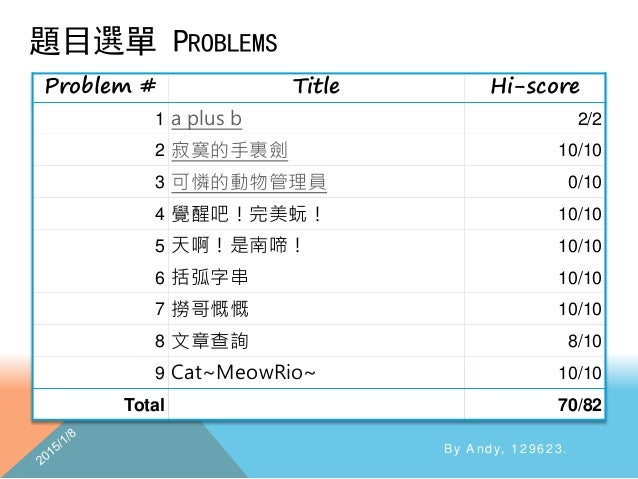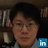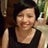Successfully reported this slideshow.You’ve finished this document.
Upcoming SlideShare
Python簡介和多版本虛擬環境架設
Next

ofUpcoming SlideShare
Python簡介和多版本虛擬環境架設
Next

Share

# Sprout 2013 Solution

The first problem solving report written by me!
Just a small test slide.

See all

See all

### Sprout 2013 Solution

1. 1. By Andy, 129623. Using C++
2. 2. 題目選單 PROBLEMS By Andy, 129623. Problem # Title Hi-score 1 a plus b 2/2 2 寂寞的手裏劍 10/10 3 可憐的動物管理員 0/10 4 覺醒吧！完美蚖！ 10/10 5 天啊！是南啼！ 10/10 6 括弧字串 10/10 7 撈哥慨慨 10/10 8 文章查詢 8/10 9 Cat~MeowRio~ 10/10 Total 70/82
3. 3. 輸 入 輸 出 By Andy, 129623. 兩個正整數a,b，中間用一空白隔開， 皆不大於10^9。 一行，a+b的值。
4. 4. CONCEPT  兩個數都不大於10^9，相加也不會大於2 × 109 ，可以用int 做就好。  int(其實是long int)可存到231 − 1 = 2147483647  除此之外好像沒有任何技巧(? By Andy, 129623.
5. 5. CODE FOR #1 By Andy, 129623. #include<iostream> using namespace std; int main(){ int a,b; cin>>a>>b; cout<<a+b<<endl; return 0; }
6. 6. 輸 入 輸 出 By Andy, 129623. 一個非負整數n（n < 1000） 輸出這n個手裏劍在完美情況下可以 造成的最大傷害值 (=個數n +安心對個數 m)
7. 7. CONCEPT  列舉觀察  n=1, m=0, ans=1  n=2, m=1, ans=3  n=3, m=3, ans=6  n=4, m=6, ans=10  難道答案是 𝑛 𝑛+1 2 ？ By Andy, 129623.
8. 8. 遞迴(RECURSION)  觀察到最優解的產生方式總是如此： By Andy, 129623. 可以證明：照著這種擺法必定有最優解。 只要考慮n<=2的特殊情形就可以了！
9. 9. CODE FOR #2 By Andy, 129623. #include<iostream> using namespace std; int main(){ int n; cin>>n; if(n<=2)cout<<n+(n*(n-1)/2)<<endl; else cout<<4*n-6<<endl; return 0; }
10. 10. 輸 入 輸 出 By Andy, 129623. 8行，每行為8個大寫英文字母 一行輸出一組輸出合法的交換，必 須排序。
11. 11. CONCEPT  想：有哪些情形可以交換？  要3個方塊相同，若且唯若原本已經 有兩個方塊相同。  像右邊這樣考慮…  垂直情形相同！  所以，對每一格，掃右和下1~2格  再來，可以寫一個函數專門來確認橘色格子是否可以移動到 藍色格子對上灰色格子，並將可以的解答記錄下來。 By Andy, 129623.
12. 12. 解答排序(SOLUTION SORTING)  大魔王來了，解答出來怎麼排序？ 1. 解答對(x1,y1)、(x2,y2)排序  注意到格子只能上下交換、左右交換  所以一定有一個座標值(x或y)是相同的！  因此，可以做一個「通吃的解法」寫成 𝑎𝑛𝑠 = min(𝑥1, 𝑥2) , min y1,y2 , min(𝑥1, 𝑥2) ,max(y1,y2) 2. 解答之間排序  我知道排序可以用sort()，可是有四個維度要怎麼排？  每個維度都是1~8，可以把它們壓成四位數再排！  輸出的時候再解開就好。  排序之後，判斷重複就方便了！ By Andy, 129623.
13. 13. CODE FOR #3 By Andy, 129623. #include<iostream> #include<algorithm> using namespace std; char t; //解答 int ans,solcnt=0; //確認並儲存(tx,ty)->(nx,ny)解答對 void check(int x,int y,int dir,int ndir){ int tx=x,ty=y; switch(dir){ case 1:tx--;ty--;break; case 2:tx--;ty++;break; case 3:tx++;ty--;break; case 4:tx++;ty++;break; case 5:tx-=2;break; case 6:ty+=2;break; case 7:tx+=2;break; case 8:ty-=2;break; } //如果不合法就退出 if(tx>8||ty>8||tx<=0||ty<=0)return; int nx=tx,ny=ty; switch(ndir){ case 1:nx--;break; case 2:nx++;break; case 3:ny--;break; case 4:ny++;break; } if(t[x][y]==t[tx][ty]){ //為解答編碼成4位數字，左上角先存 ans[solcnt++]=100*(10*min(tx,nx)+min( ty,ny))+10*max(tx,nx)+max(ty,ny); } } 注：dir: 1左上 2右上 3左下 4右下 5上x2 6右x2 7下x2 8左x2 ndir: 1上2下3左4右
14. 14. CODE FOR #3 CONT. By Andy, 129623. void sort(){ sort(ans,ans+solcnt); //避免輸重複解答 int last=ans; for(int i=1;i<solcnt;i++) if(ans[i]==last){ ans[i]=-1; }else{ last=ans[i]; } } void print(){ for(int i=0;i<solcnt;i++){ if(ans[i]==-1)continue; int from={(ans[i]/100)/10,(ans[i]/100)%10}; int to={(ans[i]%100)/10,(ans[i]%100)%10}; cout<<"("<<from<<","<<from<<"),"; cout<<"("<<to<<","<<to<<")"<<endl; } }
15. 15. CODE FOR #3 CONT. By Andy, 129623. int main(){ //先鋪1層外圍的#，表示沒有東西 for(int i=0;i<=10;i++)for(int j=0;j<=10;j++)t[i][j]='#'; //輸入 for(int i=1;i<=8;i++)for(int j=1;j<=8;j++)cin>>t[i][j]; //依序掃每一格上下左右是否有相鄰 for(int i=1;i<=8;i++){ for(int j=1;j<=8;j++){ //只要往下往右掃就可 if(t[i][j]==t[i+1][j]){ check(i,j,1,4); check(i,j,2,3); check(i+1,j,3,4); check(i+1,j,4,3); check(i,j,5,2); check(i+1,j,7,1); } if(t[i][j]==t[i+2][j]){ check(i,j,3,4); check(i,j,4,3); }
16. 16. CODE FOR #3 CONT. By Andy, 129623. if(t[i][j]==t[i][j+1]){ check(i,j,1,2); check(i,j+1,2,2); check(i,j,3,1); check(i,j+1,4,1); check(i,j,8,4); check(i,j+1,6,3); } if(t[i][j]==t[i][j+2]){ check(i,j,2,2); check(i,j,4,1); } } } sort(); print(); return 0; }
17. 17. 輸 入 輸 出 By Andy, 129623. 兩行長度相同且不超過1000個字元、 由ATCG組成的字串s1、s2。 旋轉置換一次代價為6，更改其中一 個碼代價為4，輸出最少成本使s1 成為s2。
18. 18. CONCEPT  乍看之下好像很複雜  一邊轉一邊改，還不如先轉轉看再改！  因為只能整段旋轉，其實可以直接掃：  ATCGATCG  TCGATCGA  CGATCGAT  GATCGATC …  每次轉一位，看看有幾個基因需要改，統計最小值即可！  設字串長度為n，左旋轉a次可以替換成右旋轉n-a次！  轉a位可以想成迴圈變數i加上a，字串取超過的時候從頭繼續取就好  可以用取餘數(%)完成這個操作！ By Andy, 129623.
19. 19. CODE FOR #4 (CORE FUNCTIONS) By Andy, 129623. string s1,s2; char getS1(int index){ //超過就從頭開始取 return s1[index%s1.size()]; } char getS2(int index){ //超過就從頭開始取 return s2[index%s2.size()]; }
20. 20. CODE FOR #4 CONT. By Andy, 129623. int main(){ cin>>s1>>s2; int ans=-1,len=s1.size(); for(int begin=0;begin<len;begin++){ //定義兩種操作 int opa=min(begin,len-begin),opb=0; //比較差異 for(int i=0;i<len;i++)opb+=getS1(begin+i)!=getS2(i); //更新最優解 if(ans==-1)ans=6*opa+4*opb; ans=min(ans,6*opa+4*opb); } cout<<ans; return 0; }
21. 21. 輸 入 輸 出 By Andy, 129623. 兩行字串s1、s2。 分別統計s1、s2出現X、D、r、z出 現的總次數，若s1<=s2輸出”Yes!!!”， 否則輸出”NO QAQ”。
22. 22. CONCEPT  可以寫一個函數專門做這種比對(其實也可以不用 By Andy, 129623.
23. 23. CODE FOR #5 By Andy, 129623. #include<iostream> using namespace std; bool match(char c){ return c=='X'||c=='D'||c=='r'||c=='z'; } int main(){ string a,b; int x=0,y=0; getline(cin,a); getline(cin,b); for(int i=0;i<a.size();i++)x+=match(a[i]); for(int i=0;i<b.size();i++)y+=match(b[i]); if(x<=y)cout<<"Yes!!!";else cout<<"NO QAQ"; return 0; }
24. 24. 輸 入 輸 出 By Andy, 129623. 輸入的每一行包含一個括弧字串。 同一個字串內不會有多餘空白。每 一個字串長度不超過1000000。 輸出括弧排列是否合法。
25. 25. CONCEPT  想想看自己平常是怎麼看括號合不合法？  合法：(())((()))  合法：(((()))())))  不合法：())(())()  Why? 因為它有個孤獨的右括號！  你怎麼知道它是孤獨的？  因為它前面沒有足夠的左括號！(這是關鍵)  所以，從頭開始數左括號和右括號，一旦右括號比左括號 多就不用繼續比了！  或者可以簡化：令一個變數初始為0，遇到左括號+1，遇到右括號-1， 但如果不夠減就可以停了！  最後當然要判斷左括號和右括號一不一樣多。 By Andy, 129623.
26. 26. 陷阱！  再來是這題最大的陷阱：空字串合法！可是如果只用cin讀 入空字串，根本不會處理。 By Andy, 129623.
27. 27. CODE FOR #6 By Andy, 129623. #include<iostream> using namespace std; int main(){ string s; while(getline(cin,s)){ int cnt=0,flag=1; for(int i=0;i<s.size()&&flag;i++){ if(s[i]=='(')cnt++; else { if(cnt<=0)flag=0; else cnt--; } } if(cnt)flag=0; cout<<(flag?"Yes":"No")<<endl; } }
28. 28. 輸 入 輸 出 By Andy, 129623. 輸入的第一行包含兩個整數n, k (1 ≤ n ≤ 105 , 0 ≤ k ≤ 109)，代表有n位 女生，撈網的直徑為k。 第二行包含n個整數xi (0 ≤ xi ≤ 109 )， 代表這n個女生所在的座標位置。 一個整數，為在直徑k範圍內的女生 數量
29. 29. HINT  這題觀念不難，難在要優化，否則會… By Andy, 129623.
30. 30. CONCEPT  既然只跟直徑有關，就不需要管圓心了  邊界一定要是整數，在整數點之間沒有比較好  顯然可以枚舉起點(自xi最小值到最大值)再統計區間中的女 生個數，複雜度O(n*(max(xi)-min(xi)))  在xi範圍很大時，太慢了！  還可以怎麼做？  有一個邊界一定要在女生上，否則像套橡皮筋一樣，真正能抓到女 生的範圍就變小了！  所以枚舉的起點就只有k個，複雜度降為O(kn)  統計優化：可以發現其實先把陣列排好序可以節省很多時間！  做二分搜，可以讓複雜度降為O(klogn)，不過循序已經夠好了。 By Andy, 129623.
31. 31. CODE FOR #7 By Andy, 129623. #include<iostream> #include<algorithm> using namespace std; int main(){ int n,k; cin>>n>>k; int a[n]; for(int i=0;i<n;i++)cin>>a[i]; //排序 sort(a,a+n); int ans=0; //窮舉左邊界 for(int i=0;i<n;i++){ int l=a[i],r=l+k,cnt=0; for(int j=i;j<n;j++){ if(a[j]>=l) if(a[j]<=r)cnt++; else break; } ans=cnt>ans?cnt:ans; if(r>a[n-1])break; } cout<<ans; return 0; }
32. 32. 輸 入 輸 出 By Andy, 129623. 兩行，第一行為一篇文章，以句點 為分隔，除末句外句點後面保證有 一空白。第二行為一關鍵字。 所有包含此關鍵字，且前後無多餘 字母的句子，不含行末空白。
33. 33. CONCEPT  先列出大致的流程  抓出所有句子開頭的索引值  掃這個字出現在哪  找出是在哪句出現的  如果沒輸出過，輸出那個句子(到句點為止)  接著只要一一執行就可。  小細節，比較關鍵字時要忽略大小寫  可以把它們全轉成大寫再比！ By Andy, 129623.
34. 34. CODE FOR #8 (CORE FUNCTIONS) By Andy, 129623. #include<iostream> using namespace std; //判斷是否相等，大小寫視為相同 bool isSame(char a,char b){ //先都轉換成大寫 if(b>='a'&&b<='z') b=b-'a'+'A'; if(a>='a'&&a<='z') a=a-'a'+'A'; return a==b; } bool isAlpha(char x){ return (x>='a'&&x<='z')||(x>='A'&&x<='Z'); }
35. 35. CODE FOR #8 CONT. By Andy, 129623. int main(){ int has=0; string s,tar; int strBegin,printed,sbcnt=1; getline(cin,s); cin>>tar; strBegin=printed=0; //記錄所有開始 (類似split作用) for(int i=0;i<s.size();i++) if(s[i]==‘.’){ //.->空白->下一句開頭 strBegin[sbcnt]=i+2; printed[sbcnt]=0; sbcnt++; } //末句不算，避免越界 strBegin[sbcnt--]=0; for(int i=0;i<s.size();i++){ int offset=0,flag=0; //如果首字元(前字元為-1)，前面必定 不是字母，傳回* char prevChar=(i!=0)?s[i- 1]:'*'; char nextChar=(i+tar.size() <s.size())?s[i+tar.size()]:'*'; if(!isAlpha(prevChar)&&!isAlpha(n extChar)) while(isSame(tar[offset],s[i+offs et])){ if(offset==tar.size()-1)flag=1; offset++; } else flag=0;
36. 36. CODE FOR #8 CONT. By Andy, 129623. if(flag){ has=1; int jj; for(jj=0;jj<sbcnt;jj++){ if(i<strBegin[jj])break; } if(!printed[jj]){ for(int j=strBegin[jj-1];s[j-1]!='.';j++){ cout<<s[j]; } cout<<endl; printed[jj]=1; } } } if(!has)cout<<"None."; return 0; }
37. 37. 輸 入 輸 出 By Andy, 129623. 第一行為兩個正整數n (n < 21) 與 Wmax，分別代表金幣個數以及總重 量的上限。 第二行為n個非負整數，代表這n個 金幣的重量。 最大總金幣重量。
38. 38. CONCEPT  規模不是很大(𝑛 ≤ 20)，用O(2n)再剪枝就很快了。  可以用DFS(Depth-First Search，深度優先走訪)來做，若 n的硬幣依序考慮，第k個硬幣重量Wk，且 𝑓(𝑘, 𝑊)為前k個 硬幣所能得到的最佳解，遞迴關係可以列如下： 𝑓 𝑘, 𝑊 = 0，當𝑘 = 0 0，當𝑊 < 0 max 𝑓 𝑘 − 1, 𝑊 − 𝑊𝑘 + 𝑊𝑘, 𝑓 𝑘 − 1, 𝑊 , 𝑊 ，其他情形  沒那麼複雜，其實max裡就是選與不選這兩種而已！  而其他的情形就是邊界條件(boundary condition)，你不會 希望無窮遞迴的！ By Andy, 129623.
39. 39. THINK FURTHER  其實可以DP(Dynamic Programming，動態規劃)，只不過 這題不需要而已。  請參考：0/1 Knapsack Problem@演算法筆記 By Andy, 129623.
40. 40. CODE FOR #9 By Andy, 129623. #include<iostream> using namespace std; int n,wmax,ans=0; int coin; void dfs(int dep,int sum){ if(sum>wmax)return; if(dep==n){ ans=sum>ans?sum:ans; return; } dfs(dep+1,sum+coin[dep]); dfs(dep+1,sum); } int main(){ cin>>n>>wmax; for(int i=0;i<n;i++)cin>>coin[i]; dfs(0,0); cout<<ans; }
41. 41. By Andy, 129623. Any comments or better solutions are welcome!
•#### AlinLee1

Oct. 27, 2020
•#### KevinTien2

Mar. 23, 2016
•#### wendy0751

Oct. 12, 2015

Total views

1,208

On Slideshare

0

From embeds

0

Number of embeds

3

21

Shares

0